## Abstract

The objective of the present study was to investigate the partial slip effect on an unsteady two-dimensional mixed convection stagnation point flow towards a permeable shrinking sheet. The governing equations are reduced to a system of non-dimensional partial differential equations using a semi-similarity transformation, before being solved numerically by using Keller-box method. The features of the flow characteristics for different values of the governing parameters are analysed and discussed. The results indicate that the momentum, thermal and concentration boundary layer thicknesses increase with increasing mixed convection parameter for opposing flow, whereas the opposite effect is observed for assisting flow. The results also show that the surface velocity is higher when there is slip at a sheet compared to its absent. Further, the study indicates that the boundary layer thicknesses become thicker and thicker with increasing shrinking parameter, while the opposite effect is observed with increasing Hartmann number. Comparison with previously published work for special cases is performed and found to be in excellent agreement.

## Keywords

Unsteady; Partial slip effect; MHD; Micropolar fluid; Permeable shrinking sheet

## 1. Introduction

The study of hydromagnetic stagnation point flow of an incompressible viscous fluid has attracted many researchers due to wide applications in industries and engineering. Some of the applications are the aerodynamic extrusion of plastic sheets, geothermal energy extractions, cooling of nuclear reactor, plasma studies, cooling of underground electric cables, geothermal energy extractions, artificial fibres and blood flow problems. Hiemenz  was first to investigate the two-dimensional stagnation flow towards a stationary plate. On the other hand, the flow over a linearly stretching plate was first considered by Crane . Chiam  combined Hiemenz and Crane’s problems by considering the steady two-dimensional stagnation-point flow of an incompressible viscous fluid towards a stretching sheet. Kumari and Nath  studied the effect of the magnetic field on the stagnation point flow with heat transfer over a stretching sheet. Mahapatra and Gupta  investigated the steady two-dimensional stagnation-point flow of an incompressible viscous electrically conducting fluid towards a stretching sheet. Sharma and Singh  studied the steady MHD two-dimensional stagnation point flow towards a stretching sheet with variable thermal conductivity. Ashraf and Rashid  investigated the radiation effect on MHD two-dimensional stagnation point flow towards a heated shrinking sheet. Mostafa and Shimaa  investigated the combined effects of magnetic field and thermal radiation on a steady micropolar fluid near a stagnation point towards a moving surface. Bhattacharyya et al.  studied the boundary layer flow near a stagnation point with heat and mass transfer over a shrinking sheet with Soret and Dufour effects. Zeeshan et al.  investigated MHD natural convection flow of a water/ethylene glycol nanofluid with inverted cone through a porous medium. Ellahi et al.  examined MHD flow of a Jeffrey fluid on a peristaltic flow in a rectangular duct embedded in a porous medium. Khan et al.  investigated the peristaltic motion and heat transfer of Oldroyd fluid in a channel with inclined magnetic field. The effect of magnetic field on blood flow of Prandtl fluid in a porous walls through a tapered stenosed artery was investigated by Ellahi et al. . Akbar et al.  studied the combined effects of magnetic field and heat flux for two different water based nanoparticles for the peristaltic flow in a symmetric vertical permeable channel. Recently, Kandelousi and Ellahi  examined the combined effects of MHD and ferrohydrodynamic on Fe3O4-plasma nanofluid flow in a vessel and obtained the results using lattice Boltzmann method.

In recent times, the boundary layer flow due to a shrinking sheet is received considerable attention due to its wide engineering applications. Miklavčič and Wang  studied the steady viscous flow over a shrinking sheet for both two-dimensional and axisymmetric cases. The effect of suction on MHD boundary layer flow with heat and mass transfer past a shrinking sheet was examined by Muhaimin et al. . Fang and Zhang  obtained an exact solution for steady MHD flow with suction over a shrinking sheet. Since the vorticity (rotation or non-potential) flow over a shrinking sheet is not confined within the boundary layer and the flow is likely to exist, whether an adequate suction on the boundary is imposed or a stagnation point (which contains the vorticity) is considered. The stagnation point flow towards a shrinking sheet for both two-dimensional and axisymmetric cases was first investigated by Wang . He found that solutions do not exist for large shrinking rates and may be non-unique in two-dimensional case. Fang et al.  numerically investigated the viscous flow over an unsteady shrinking sheet with mass transfer. Fan et al.  extended Wang’s problem to unsteady flow. Suali et al.  studied heat transfer on an unsteady stagnation point flow with suction over a stretching/shrinking sheet. Recently, Bhattacharyya  discussed the unsteady boundary layer flow and heat transfer near a stagnation-point towards a shrinking/stretching sheet.

All of the above mentioned studies are restricted to the flows of Newtonian fluids. These Newtonian fluids cannot describe some engineering and industrial processes which are made up of materials having an internal structure. The micropolar fluids are described as a non-Newtonian fluid consisting of dumb-bell molecule, fluid suspension, polymer fluids, animal blood. The presence of smoke or dust in a gas may also model using micropolar fluid dynamics. The theory of micropolar fluid model introduced by Eringen  exhibits the local effects arising from the microstructure and micro motion of the fluid elements. Comprehensive reviews of the subject and its applications can be found in the review articles of Peddieson and McNitt , Ariman et al.  and  and books by Łukaszewicz  and Eringen . Guram and Smith  and Gorla  investigated the steady micropolar fluid near a stagnation point. Nazar et al.  examined the steady two-dimensional stagnation-point flow over a stretching sheet. Ishak et al.  studied the two-dimensional mixed convection boundary layer flow of a micropolar fluid near the stagnation point over a stretching sheet. Ishak et al.  numerically investigated the steady two-dimensional stagnation-point flow of a micropolar fluid over a shrinking sheet. Ashraf and Bashir  numerically investigated the effect of MHD on the steady two-dimensional stagnation-point flow of a micropolar fluid towards a heated shrinking sheet. Aman et al.  studied the mixed convection stagnation point flow on a vertical surface in the presence of slip effects. Reddy et al.  considered the boundary layer flow near a stagnation point of electrically conducting micropolar fluid over a stretching sheet with viscous dissipation, chemical reaction and heat source/sink. Hayat et al.  examined the unsteady stagnation point flow of second grade fluid over a porous stretching sheet. Ellahi et al.  investigated the unsteady flow of a micropolar fluid through a composite stenosis in arterial blood flow with slip velocity. In other paper, Ellahi et al.  studied micropolar fluid with heat and mass transfer of blood flow in a porous walls through a tapered stenosis arteries. Recently, Aurangzaib and Shafie  investigated the unsteady MHD flow near a stagnation-point of a micropolar fluid past a shrinking sheet with thermophoresis and slip effects.

In some practical situations, it is important to replace the no-slip condition by the partial slip condition because when fluid flows in micro electro mechanical system (MEMS), the no-slip boundary condition at the solid-fluid interface is no longer applicable. The non-equilibrium region near the interface is more accurately described by the slip flow model. Wang  found closed form solution of two-dimensional viscous flow due to stretching sheet with the effects of slip and suction. Aman and Ishak  investigated the effect of partial slip on the steady incompressible flow over shrinking permeable sheet. Das  studied the effect of partial slip and temperature dependent fluid properties on steady hydro-magnetic micropolar fluid flow over an inclined permeable plate in the presence of chemical reaction, thermal radiation and heat source/sink. Das  investigated the steady MHD mixed convection boundary layer stagnation point flow of a micropolar fluid towards a shrinking sheet in the presence of partial slip. Ellahi and Hameed  investigated MHD flow of a second grade fluid with heat transfer in a channel with nonlinear slip effects. Zeeshan and Ellahi  obtained the series solution of MHD slip flow and heat transfer of a non-Newtonian third grade fluid in a porous pipe. The mixed convection flow and heat transfer past a porous vertical cylinder were investigated by Ellahi et al. . Recently, Chen  considered the unsteady mixed convection stagnation point flow on a stretching sheet with slip effect.

Motivated by the above discussions, the effect of partial slip on an unsteady MHD mixed convection stagnation point flow with heat and mass transfer in a micropolar fluid towards a permeable shrinking sheet is investigated in this paper. The unsteadiness is caused by the impulsive motion of the external stream or of the shrinking surface. The governing equations are solved numerically by using Keller-box method. The obtained results are then compared with previous published results available in the literature.

## 2. Mathematical formulations

Consider an unsteady two-dimensional mixed convection stagnation point flow of an incompressible electrically conducting micropolar fluid towards a permeable shrinking sheet. A transverse magnetic field ${\textstyle B_{0}}$ is applied in the y-direction and induced magnetic field is negligibly assumed to be very small magnetic Reynolds number. The flow configuration is shown schematically in Fig. 1. It is assumed that the velocity ${\textstyle u_{w}(x)}$, the temperature ${\textstyle T_{w}(x)}$ and the concentration ${\textstyle C_{w}(x)}$ of the sheet are proportional to the distance ${\textstyle x}$ from the stagnation point, where ${\textstyle T_{w}(x)>T_{\infty }}$ and ${\textstyle C_{w}(x)>C_{\infty }}$ with ${\textstyle T_{\infty }}$ and ${\textstyle C_{\infty }}$ being the uniform temperature and concentration of the ambient fluid. The velocity of the flow external to the boundary layer is ${\textstyle u_{e}(x)}$. Under the usual boundary layer approximation, along with Boussinesq approximations the governing equations are governed by the following equations:

 ${\frac {\partial u}{\partial x}}+{\frac {\partial v}{\partial y}}=$$0{\mbox{,}}$
(1)
 ${\frac {\partial u}{\partial t}}+u{\frac {\partial u}{\partial x}}+$$v{\frac {\partial u}{\partial y}}=u_{e}{\frac {{du}_{e}}{dx}}+$$\left({\frac {\mu +\kappa }{\rho }}\right){\frac {{\partial }^{2}u}{\partial y^{2}}}+$${\frac {\kappa }{\rho }}{\frac {\partial N}{\partial y}}+$$g\beta (T-T_{\infty })+g\beta {_{\ast }}(C-C_{\infty })+$${\frac {\sigma B_{0}^{2}}{\rho }}(u_{e}-u){\mbox{,}}$
(2)
 ${\frac {\partial N}{\partial t}}+u{\frac {\partial N}{\partial x}}+$$v{\frac {\partial N}{\partial y}}={\frac {\gamma }{\rho j}}{\frac {{\partial }^{2}N}{\partial y^{2}}}-$${\frac {\kappa }{\rho j}}\left(2N+{\frac {\partial u}{\partial y}}\right){\mbox{,}}$
(3)
 ${\frac {\partial T}{\partial t}}+u{\frac {\partial T}{\partial x}}+$$v{\frac {\partial T}{\partial y}}={\frac {k}{\rho c_{p}}}{\frac {{\partial }^{2}T}{\partial y^{2}}}{\mbox{,}}$
(4)
 ${\frac {\partial C}{\partial t}}+u{\frac {\partial C}{\partial x}}+$$v{\frac {\partial C}{\partial y}}=D{\frac {{\partial }^{2}C}{\partial y^{2}}}{\mbox{,}}$
(5)

where ${\textstyle \left(u{\mbox{,}}v\right)}$ are the velocity components along the ${\textstyle \left(x{\mbox{,}}y\right)}$ axes, respectively, ${\textstyle c_{p}}$ is the specific heat at constant pressure, ${\textstyle \kappa }$ is the vortex viscosity, ${\textstyle \sigma }$ is the electrical conductivity of the fluid, ${\textstyle B_{0}}$ is externally imposed magnetic field strength in the y  -direction, ${\textstyle \rho }$ is the density of the fluid, ${\textstyle D}$ is the molecular diffusivity, ${\textstyle k}$ is the coefficient of thermal expansion, ${\textstyle N}$ is the components of microrotation or angular velocity whose rotation is in the direction of the ${\textstyle x{\mbox{,}}y}$ – planes, ${\textstyle \gamma }$ is the spin gradient viscosity given by ${\textstyle \gamma =(\mu +\kappa /2)j=\mu (1+K/2)j}$, ${\textstyle j}$ is the micro-inertia density, and ${\textstyle \mu }$ is the coefficient of viscosity of the fluid. According to Xu  we assume that ${\textstyle j}$ is constant therefore ${\textstyle j=\upsilon /a}$.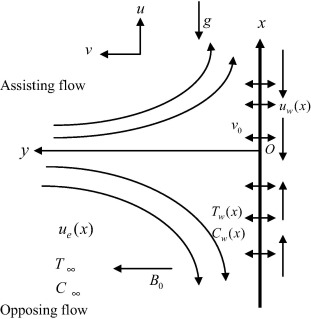Figure 1. Physical model and coordinate system.

The appropriate physical boundary conditions for the problem under study are given by

 $t<0:\quad u(t{\mbox{,}}x{\mbox{,}}y)=0{\mbox{,}}v(t{\mbox{,}}x{\mbox{,}}y)=$$0{\mbox{,}}N(t{\mbox{,}}x{\mbox{,}}y)=0{\mbox{,}}T(t{\mbox{,}}x{\mbox{,}}y)=$$T_{\infty }{\mbox{,}}C(t{\mbox{,}}x{\mbox{,}}y)=C_{\infty }\quad {\mbox{for any}}\quad x{\mbox{,}}y{\mbox{,}}$

 $t\geqslant 0:\quad u=bx+L{\frac {\partial u}{\partial y}}{\mbox{,}}v=$$v_{0}{\mbox{,}}N=-n{\frac {\partial u}{\partial y}}{\mbox{,}}T=$$T_{w}{\mbox{,}}C=C_{w}\quad {\mbox{at}}\quad y=0{\mbox{,}}$
(6)
 $u\rightarrow u_{e}(x){\mbox{,}}N\rightarrow 0{\mbox{,}}T\rightarrow T_{\infty }{\mbox{,}}C\rightarrow C_{\infty }\quad {\mbox{as}}\quad \quad y\rightarrow \infty {\mbox{.}}$

The sheet velocity ${\textstyle u_{w}(x)}$, temperature ${\textstyle T_{w}(x)}$ and concentration ${\textstyle C_{w}(x)}$ are assumed to vary proportional to the distance ${\textstyle x}$ from the stagnation point and the velocity of the flow external to the boundary layer is ${\textstyle u_{e}(x)}$ are taken of the form:

 $u_{w}(x)=bx{\mbox{,}}\quad T_{w}(x)=T_{\infty }+cx{\mbox{,}}C_{w}(x)=$$C_{\infty }+dx\quad {\mbox{and}}\quad u_{e}(x)=ax{\mbox{.}}$
(7)

where ${\textstyle a{\mbox{,}}b{\mbox{,}}c}$ and ${\textstyle d}$ are positive constants, and ${\textstyle n}$ is a constant such that ${\textstyle 0\leqslant n\leqslant 1}$. It is noted the case when ${\textstyle n=0}$ is called a strong concentration. In this case, ${\textstyle N=0}$ near the wall implying that the concentrated particle flows in which the micro-elements close to the wall surface are unable to rotate . The case ${\textstyle n=1/2}$ presents the vanishing of the anti-symmetric part of the stress tensor and denotes weak concentrations whereas ${\textstyle n=1}$ is used for modelling of turbulent boundary layer flows. In this study, we only consider strong concentration ${\textstyle \left(n=0\right)}$.

Introducing the following non-dimensional variables as

 $\psi ={\sqrt {a\upsilon }}\xi xf(\xi {\mbox{,}}\eta ){\mbox{,}}\eta =$${\sqrt {\frac {a}{\upsilon }}}{\frac {y}{\xi }}{\mbox{,}}N=$${\sqrt {\frac {a^{3}}{\upsilon }}}{\frac {x}{\xi }}h(\xi {\mbox{,}}\eta ){\mbox{,}}$ $\xi ={\sqrt {1-e^{-4\tau }}}{\mbox{,}}\tau =at{\mbox{,}}\theta (\xi {\mbox{,}}\eta )=$${\frac {\left(T-T_{\infty }\right)}{\left(T_{w}-T_{\infty }\right)}}{\mbox{,}}\phi (\xi {\mbox{,}}\eta )=$${\frac {\left(C-C_{\infty }\right)}{\left(C_{w}-C_{\infty }\right)}}{\mbox{.}}$
(8)

where ${\textstyle 0<\xi \leqslant 1}$ and the continuity Eq. (1) is satisfied by introducing a stream function ${\textstyle \psi }$ such that

 $u={\frac {\partial \psi }{\partial y}}{\mbox{,}}\quad v=$$-{\frac {\partial \psi }{\partial x}}{\mbox{.}}$

The transformed nonlinear partial differential equations are as follows:

 $(1+K)f_{\eta \eta \eta }+2\eta (1-{\xi }^{2})f_{\eta \eta }+$${Kh}_{\eta }+{\xi }^{2}\left(1+{ff}_{\eta \eta }-f_{\eta }^{2}+\right.$$\left.M^{2}(1-f_{\eta })+\lambda (\theta +\delta \phi )\right)=$$2\xi (1-{\xi }^{2}){\frac {\partial f_{\eta }}{\partial \xi }}{\mbox{,}}$
(9)

 $(1+K/2)h_{\eta \eta }+2(1-{\xi }^{2})(h+\eta h_{\eta })+$${\xi }^{2}\left({fh}_{\eta }-f_{\eta }h-K(2h+f_{\eta \eta })\right)=$$2\xi (1-{\xi }^{2}){\frac {\partial h}{\partial \xi }}{\mbox{,}}$
(10)

 ${\frac {1}{Pr}}{\theta }_{\eta \eta }+2\eta (1-{\xi }^{2}){\theta }_{\eta }+$${\xi }^{2}(f{\theta }_{\eta }-f_{\eta }\theta )=2\xi (1-$${\xi }^{2}){\frac {\partial \theta }{\partial \xi }}{\mbox{,}}$
(11)

 ${\frac {1}{Sc}}{\phi }_{\eta \eta }+2\eta (1-{\xi }^{2}){\phi }_{\eta }+$${\xi }^{2}(f{\phi }_{\eta }-f_{\eta }\phi )=2\xi (1-$${\xi }^{2}){\frac {\partial \phi }{\partial \xi }}{\mbox{,}}$
(12)

subject to the boundary conditions (6) which become

 $f(\xi {\mbox{,}}0)={\frac {f_{0}}{\xi }}{\mbox{,}}f_{\eta }(\xi {\mbox{,}}0)=$$\epsilon +{\frac {\alpha }{\xi }}f_{\eta \eta }(\xi {\mbox{,}}0){\mbox{,}}h(\xi {\mbox{,}}0)=$$-{nf}_{\eta \eta }(\xi {\mbox{,}}0){\mbox{,}}\theta (\xi {\mbox{,}}0)=$$1{\mbox{,}}\phi (\xi {\mbox{,}}0)=1{\mbox{,}}$ $f_{\eta }(\xi {\mbox{,}}\eta )\rightarrow 1{\mbox{,}}h(\xi {\mbox{,}}\eta )\rightarrow 0{\mbox{,}}\theta (\xi {\mbox{,}}\eta )\rightarrow 0{\mbox{,}}\phi (\xi {\mbox{,}}\eta )\rightarrow 0\quad {\mbox{as}}\quad \eta \rightarrow \infty {\mbox{.}}$
(13)

where ${\textstyle f_{0}=-V_{w}/{\sqrt {a\upsilon }}}$ is the suction ${\textstyle \left(f_{0}>0\right)}$ or injection ${\textstyle \left(f_{0}<0\right)}$ parameter, ${\textstyle M^{2}=B_{0}{\sqrt {\sigma /\rho a}}}$ is the Hartmann number, ${\textstyle \lambda ={Gr}_{x}/{Re}_{x}^{2}}$ is the mixed convection parameter, ${\textstyle {Gr}_{x}=\left[g\beta (T_{w}-T_{\infty })x^{3}/{\upsilon }^{2}\right]}$ is the local Grashof number, ${\textstyle {Re}_{x}=u_{w}x/\upsilon }$ is the local Reynolds number, ${\textstyle \delta =\left[{\beta }^{_{\ast }}(C_{w}-C_{\infty })/\beta (T_{w}-\right.}$$\left.T_{\infty })\right]$ is the constant concentration buoyancy parameter, ${\textstyle Pr=\mu c_{p}/k}$ is the Prandtl number, ${\textstyle \alpha =L{\sqrt {a/\upsilon }}}$ is the slip parameter, ${\textstyle Sc=\upsilon /D}$ is Schmidt number, and ${\textstyle \epsilon =b/a}$ with ${\textstyle \epsilon >0}$ and ${\textstyle \epsilon <0}$ correspond to a stretching and shrinking sheet, respectively. It should be noted that dual solutions is only possible when ${\textstyle \left(\xi =1\right)}$ which can be seen in Figure 17, Figure 18, Figure 19, Figure 2 and Figure 20.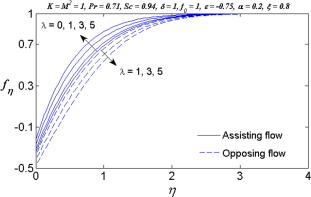Figure 2. Variation of ${\textstyle \lambda }$ on the velocity ${\textstyle f_{\eta }}$.

The quantities of physical interest are the skin-friction coefficient in the x  -direction ${\textstyle C_{fx}{\mbox{,}}}$ and the Nusselt number ${\textstyle {Nu}_{x}}$ and the Sherwood number ${\textstyle {Sh}_{x}}$ are defined respectively by,

 $C_{fx}={\frac {{\tau }_{w}}{\rho u_{e}^{2}}}{\mbox{,}}{\tau }_{w}=$${\left[(\mu +\kappa ){\frac {\partial u}{\partial y}}+\kappa N\right]}_{y=0}\quad {\mbox{then}}\quad C_{fx}{Re}_{x}^{\frac {1}{2}}=$${\frac {1}{\xi }}[1+(1-n)K]f_{\eta \eta }(\xi {\mbox{,}}0){\mbox{,}}$
 $q_{w}=-k{\left({\frac {\partial T}{\partial y}}\right)}_{y=0}{\mbox{,}}{Nu}_{x}=$${\frac {q_{w}}{\left(T_{w}-T_{\infty }\right)}}\left({\frac {x}{k}}\right)\quad {\mbox{then}}\quad {Nu}_{x}/{Re}_{x}^{\frac {1}{2}}=$$-{\frac {1}{\xi }}{\theta }_{\eta }(\xi {\mbox{,}}0){\mbox{,}}$
 $m_{w}=-\rho D{\left({\frac {\partial C}{\partial y}}\right)}_{y=0}{\mbox{,}}{Sh}_{x}=$${\frac {m_{w}}{\left(C_{w}-C_{\infty }\right)}}\left({\frac {x}{\rho D}}\right)\quad {\mbox{then}}\quad {Sh}_{x}/{Re}_{x}^{\frac {1}{2}}=$$-{\frac {1}{\xi }}{\phi }_{\eta }(\xi {\mbox{,}}0){\mbox{.}}$

## 3. Numerical method

The nonlinear partial differential Eqs. (9), (10), (11) and (12) subject to boundary conditions (13) were solved numerically using an implicit finite difference scheme, known as the Keller-box method. This method has been found to be very suitable in dealing with nonlinear parabolic problems. By introducing the new dependent variables, these equations are first written as a system of first-order equations which are then expressed in finite difference forms using central differences. Since the system of equations is nonlinear, it is linearized using Newton’s method before putting them in matrix-vector form. The resulting linear system is solved along with the boundary conditions by the block-tridiagonal-elimination method. Details of the method can be found in the book by Cebeci and Bradshaw . In this study, we used MATLAB software. Here, the value of step size ${\textstyle \Delta \eta =0.01}$ has been used and the convergence criterion was set to ${\textstyle 5\times {10}^{-5}}$, which give accuracy to four decimal places. The satisfaction of the outer boundary condition is achieved by considering the boundary layer thickness ${\textstyle {\eta }_{\infty }=10}$. However for dual solutions, we considered different boundary layer thicknesses ${\textstyle {\eta }_{\infty }}$. The correctness of the present numerical scheme, a comparison of the obtained numerical results corresponding to the skin friction coefficient ${\textstyle f^{''}(0)}$ is checked with the previous published results of Wang  and Ishak et al.  in Table 1. Thus, it is seen from Table 1 that the numerical results are in close agreement.

Table 1. Values of ${\textstyle f^{''}(0)}$ for stretching sheet in the absence of buoyancy force ${\textstyle \phi }$, Hartmann number ${\textstyle M^{2}}$ and slip parameter ${\textstyle \alpha }$.
${\textstyle \epsilon }$ Wang  Ishak et al.  Present ${\textstyle \left(\xi =1\right)}$
${\textstyle K=0}$ ${\textstyle K=1{\mbox{,}}n=0.5}$ ${\textstyle K=0}$ ${\textstyle K=1{\mbox{,}}n=0.5}$
0 1.23258 1.232588 1.006404 1.232588 1.006405
0.1 1.14656 1.146561 0.936163 1.146562 0.936162
0.2 1.05113 1.051130 0.858244 1.051131 0.858246
0.5 0.71330 0.713295 0.582403 0.713300 0.582403
1 0 0 0 0 0
2 −1.88731 −1.887307 −1.540979 −1.887307 −1.540980
3 −4.276541 −3.491781 −4.276540 −3.491782
4 −7.086378 −5.786003 −7.086380 −5.786005
5 −10.26475 −10.264749 −8.381133 −10.264750 −8.381132

## 4. Results and discussion

Figure 2, Figure 20, Figure 3, Figure 4 and Figure 5 illustrate the effects for the mixed convection parameter ${\textstyle \lambda }$ on the velocity, microrotation, temperature and concentration of the fluid for both assisting and opposing flows. From Figure 2 and Figure 3, it is observed that the velocity and microrotation profiles decrease for opposing flow and it makes the momentum and microrotation boundary layer thicknesses thicker and thicker, while the reverse trend is observed for the assisting flow. Negative values of velocity close to the sheet indicate the region of reverse flow. This is due to the fact that an increase in the value of ${\textstyle \lambda }$ leads to an increase in the temperature difference ${\textstyle \left(T_{w}-T_{\infty }\right)}$. This leads to an increase in the convection currents as a result of which fluid velocity increases and thus, boundary layer thickness decreases in case of assisting flow. It is also observed from Fig. 3 that the microrotation profile is negative near the sheet and it gradually increases, i.e. accelerates the fluid far away from the sheet. The temperature of the fluid decreases with an increase in the value of mixed convection parameter until it achieves a constant value, namely zero for the assisting flow as shown in Fig. 4, whereas the opposite trend is observed for the opposing flow. This is not surprising since the fluid receives the heat from the surface and then the heat energy changed into other forms such as kinetic energy. The concentration boundary layer thickness decreases with increasing mixed convection parameter for the assisting flow as shown in Fig. 5, while the reverse trend is observed for the case of opposing flow. It is evident from these figures that all curves approach the far field boundary conditions (13) asymptotically.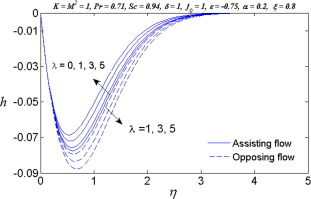Figure 3. Variation of ${\textstyle \lambda }$ on the microrotation ${\textstyle h}$.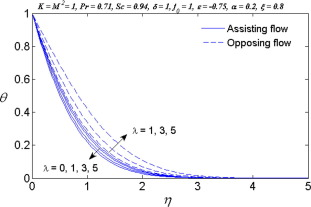Figure 4. Variation of ${\textstyle \lambda }$ on the temperature ${\textstyle \theta }$.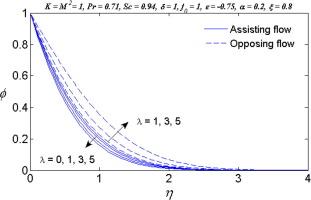Figure 5. Variation of ${\textstyle \lambda }$ on the concentration ${\textstyle \phi }$.

Figure 6, Figure 7, Figure 8 and Figure 9 illustrate the effect of shrinking parameter ${\textstyle \epsilon }$ on the velocity, microrotation, temperature and concentration profiles. It is observed from Figure 6 and Figure 7 that the velocity and microrotation profiles decrease with an increase in the value of magnitude of shrinking parameter ${\textstyle \epsilon }$ and consequently the thicknesses of momentum and microrotation boundary layer increases. Reverse flow is observed near the surface as the magnitude of shrinking parameter increases. On the other hand, the temperature and concentration of fluid increase with an increase in the value of magnitude of shrinking parameter ${\textstyle \epsilon }$ as shown in Figure 8 and Figure 9. Thus, the thermal and concentration boundary layer thicknesses become thicker and thicker.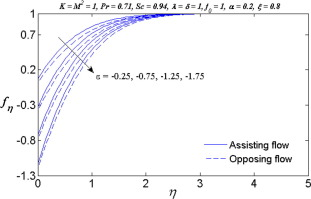Figure 6. Variation of ${\textstyle \epsilon }$ on the velocity ${\textstyle f_{\eta }}$.Figure 7. Variation of ${\textstyle \epsilon }$ on the microrotation ${\textstyle h}$.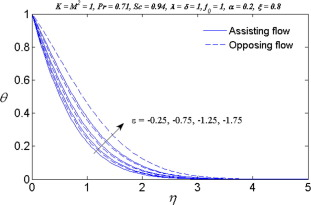Figure 8. Variation of ${\textstyle \epsilon }$ on the temperature ${\textstyle \theta }$.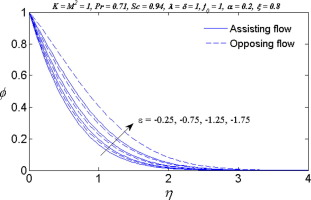Figure 9. Variation of ${\textstyle \epsilon }$ on the concentration ${\textstyle \phi }$.

The influence of Hartmann number ${\textstyle M^{2}}$ on the velocity, microrotation, temperature and concentration profiles is displayed in Figure 10, Figure 11, Figure 12 and Figure 13 in the presence of slip and in the absence of slip at the boundary. From Figure 10 and Figure 11, it is observed that for both slip and no-slip cases, the velocity and microrotation profiles along the sheet increase with increasing Hartmann number and it makes the momentum and microrotation boundary layer thicknesses thinner and thinner. This is due to the fact that the magnetic force enhances the fluid motion in the boundary layer because of the last term of momentum equation ${\textstyle \left(u_{\infty }-u\right)}$ remains positive in the boundary layer region. Here an electrically conducting fluid produces a drag-like force called the Lorentz force associated with the magnetic field makes the boundary layer thinner. As expected, the velocity and microrotation profiles are lower for the case of no-slip than those for the presence of slip. Figure 12 and Figure 13 show that the temperature and concentration of fluid decrease with increasing Hartmann number for slip as well as for no-slip conditions and cause to decrease the thermal and concentration boundary layer thicknesses. On the other hand, in the presence of slip, the temperature and concentration of fluid are lower compared to the case of no-slip. This means that the heat and mass are transferred from hot surface to the cool fluid.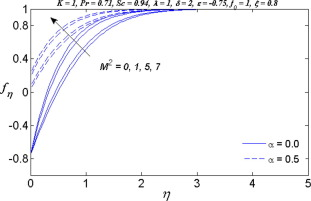Figure 10. Variation of ${\textstyle M^{2}}$ and ${\textstyle \alpha }$ on the velocity ${\textstyle f_{\eta }}$.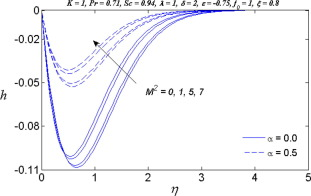Figure 11. Variation of ${\textstyle M^{2}}$ and ${\textstyle \alpha }$ on the microrotation ${\textstyle h}$.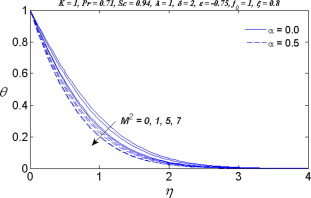Figure 12. Variation of ${\textstyle M^{2}}$ and ${\textstyle \alpha }$ on the temperature ${\textstyle \theta }$.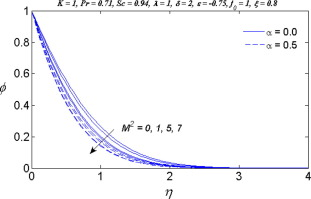Figure 13. Variation of ${\textstyle M^{2}}$ and ${\textstyle \alpha }$ on the concentration ${\textstyle \phi }$.

Figure 14, Figure 15 and Figure 16 demonstrate the effect of material parameter ${\textstyle K}$ on the reduced skin friction, the reduced Nusselt number and the reduced Sherwood number for suction or injection. It is observed from these results that the reduced skin friction, reduced Nusselt number and reduced Sherwood number decrease with an increase in the value of micropolar parameter ${\textstyle K}$. This discloses the fact that the micropolar fluids offer great resistance to the fluid motion as compared with Newtonian fluid. This phenomenon also reflects the fact that the increase in the value of micropolar parameter results in an enhancement of the total viscosity in the fluid flow. Therefore, micropolar fluid is a very efficient fluid medium in boundary layer for controlling the laminar flow. It is also observed from these figures that the suction parameter enhances the reduced skin friction, the reduced Nusselt number and the reduced Sherwood number as shown in Table 2. Opposite effect is observed for injection. Further, it is seen from these results that the value of ${\textstyle f^{''}(\xi {\mbox{,}}0){\mbox{,}}-{\theta }^{'}(\xi {\mbox{,}}0)}$ and ${\textstyle -{\phi }^{'}(\xi {\mbox{,}}0)}$ is always positive, which implies that the fluid exerts a drag force on the sheet and heat and mass are transferred from hot sheet to cold fluid, respectively.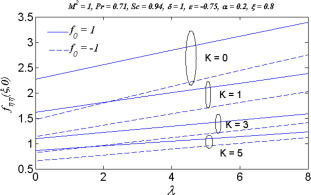Figure 14. Variation of ${\textstyle K}$ and ${\textstyle f_{0}}$ on the reduced skin friction versus ${\textstyle \lambda }$.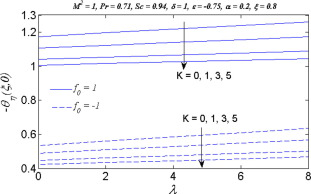Figure 15. Variation of ${\textstyle K}$ and ${\textstyle f_{0}}$ on the reduced Nusselt number versus ${\textstyle \lambda }$.Figure 16. Variation of ${\textstyle K}$ and ${\textstyle f_{0}}$ on the reduced Sherwood number versus ${\textstyle \lambda }$.

Table 2. Computed values of the reduced skin friction, reduced Nusselt number and reduced Sherwood number for various values ${\textstyle f_{0}{\mbox{,}}\lambda }$ and ${\textstyle K}$ when ${\textstyle M^{2}=1{\mbox{,}}\delta =1{\mbox{,}}Sc=0.94{\mbox{,}}}$${\textstyle Pr=0.71{\mbox{,}}\epsilon =-0.75}$${\textstyle \alpha =0.2{\mbox{,}}\xi =0.8}$.
${\textstyle f_{0}}$ ${\textstyle \lambda }$ ${\textstyle K}$ ${\textstyle f_{\eta \eta }(\xi {\mbox{,}}0)}$ ${\textstyle -{\theta }_{\eta }(\xi {\mbox{,}}0)}$ ${\textstyle -{\phi }_{\eta }(\xi {\mbox{,}}0)}$
−1 0 0 1.469512 0.532935 0.562585
1 1.133350 0.489613 0.514304
3 0.816596 0.446069 0.466404
2 0 1.799685 0.559284 0.592588
1 1.362586 0.509448 0.536666
3 0.968109 0.460173 0.482152
1 0 0 2.264289 1.172826 1.397221
1 1.616277 1.105574 1.318972
3 1.097223 1.040262 1.244493
2 0 2.555103 1.195132 1.423502
1 1.810903 1.122571 1.338763
3 1.221325 1.052368 1.258434

Figure 17, Figure 18, Figure 19, Figure 2 and Figure 20 show the dual velocity, microrotation, temperature and concentrations for different values of ${\textstyle K}$. Examining the dual velocity profiles (Fig. 17) for different values of ${\textstyle K}$, it reveals that due to the material parameter, the momentum boundary layer thickness increases for the first solution and reduces for the second solution. On the other hand, the microrotation profiles decrease with increasing ${\textstyle K}$ for both first and second solutions as shown in Fig. 18. From Figure 19 and Figure 20, it is found that the temperature and concentration of fluid increase with ${\textstyle K}$ for both solutions. Further, the second solution exhibits a larger boundary layer thickness compared to the first solution.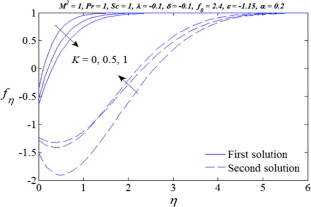Figure 17. Variation of ${\textstyle K}$ on the velocity ${\textstyle f_{\eta }}$.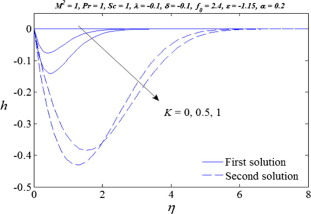Figure 18. Variation of ${\textstyle K}$ on the microrotation ${\textstyle h}$.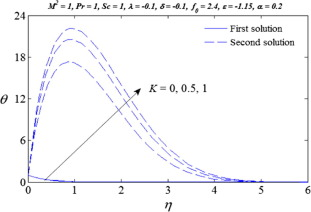Figure 19. Variation of ${\textstyle K}$ on the temperature ${\textstyle \theta }$.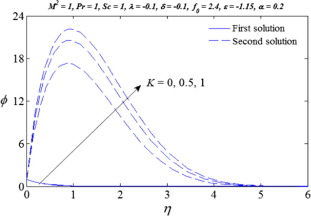Figure 20. Variation of ${\textstyle K}$ on the concentration ${\textstyle \phi }$.

## 5. Conclusions

The combined effects of heat and mass transfer on an unsteady MHD mixed stagnation point flow of a micropolar fluid towards a permeable shrinking sheet in the presence of slip effect are investigated. The numerical results were compared with those from previous studied to verify their validity. In the light of the present investigation, it is found that the momentum, microrotation, thermal and concentration boundary layer thicknesses increase with increasing mixed convection parameter for opposing flow, whereas reverse effect is observed for assisting flow. In addition, the momentum, microrotation, thermal and concentration boundary layer thicknesses decrease with an increase in the value of Hartmann number for both cases of slip and no-slip conditions. Further, the shrinking parameter enhanced the momentum, thermal and concentration boundary layer thicknesses. Furthermore, the dual solutions is only possible for when ${\textstyle \xi =1}$.

## Acknowledgement

We would like to express our very sincerely thanks to the reviewers for very useful suggestions to improve the version of the paper. The first author would like to acknowledge the Dean of Science for the financial support for this research.

### Document informationPublished on 12/04/17

Licence: Other

### Document Score0

Views 29
Recommendations 0